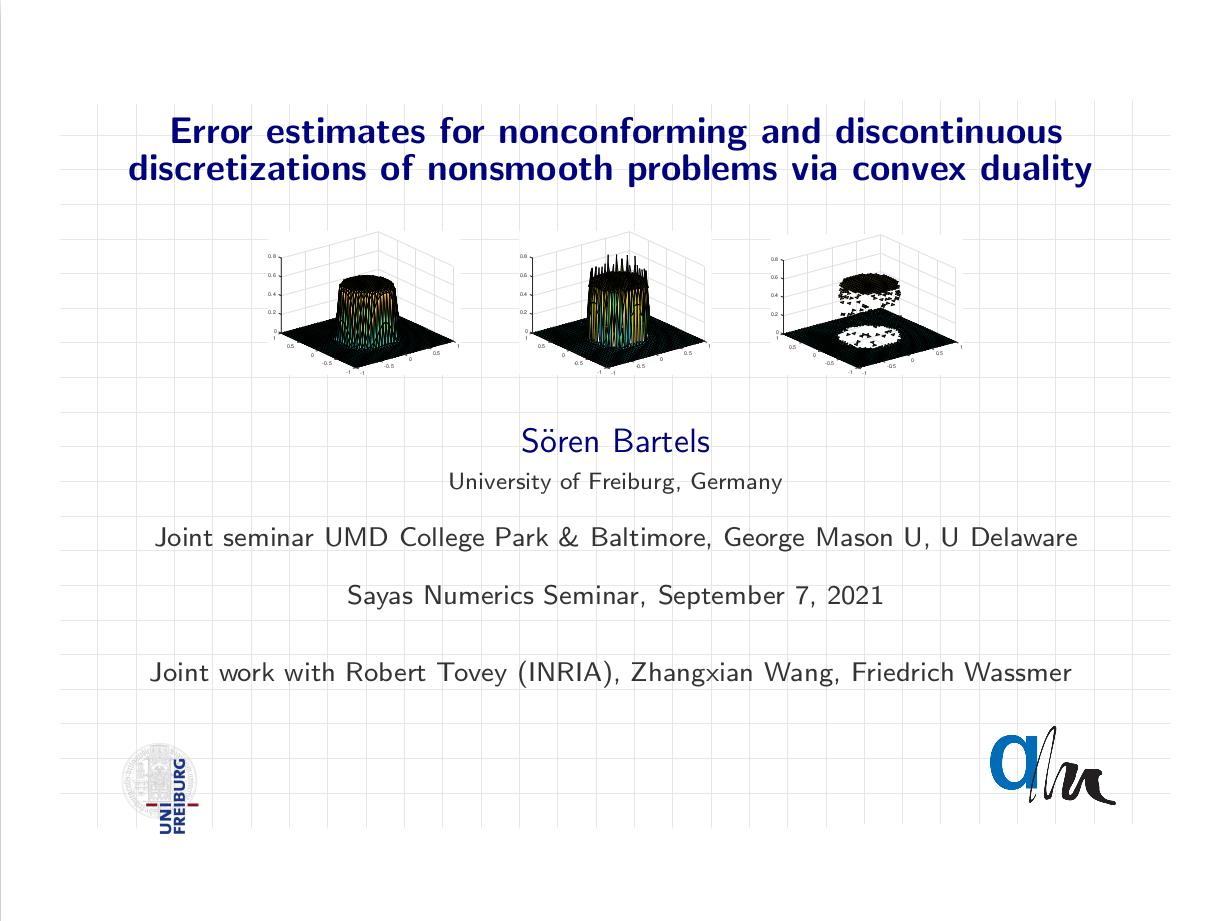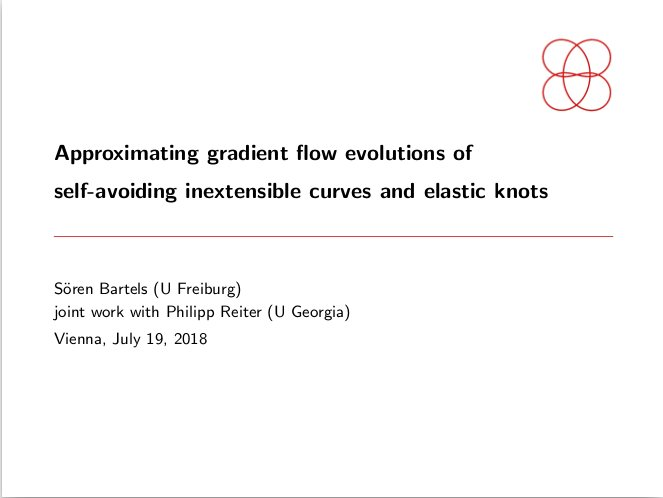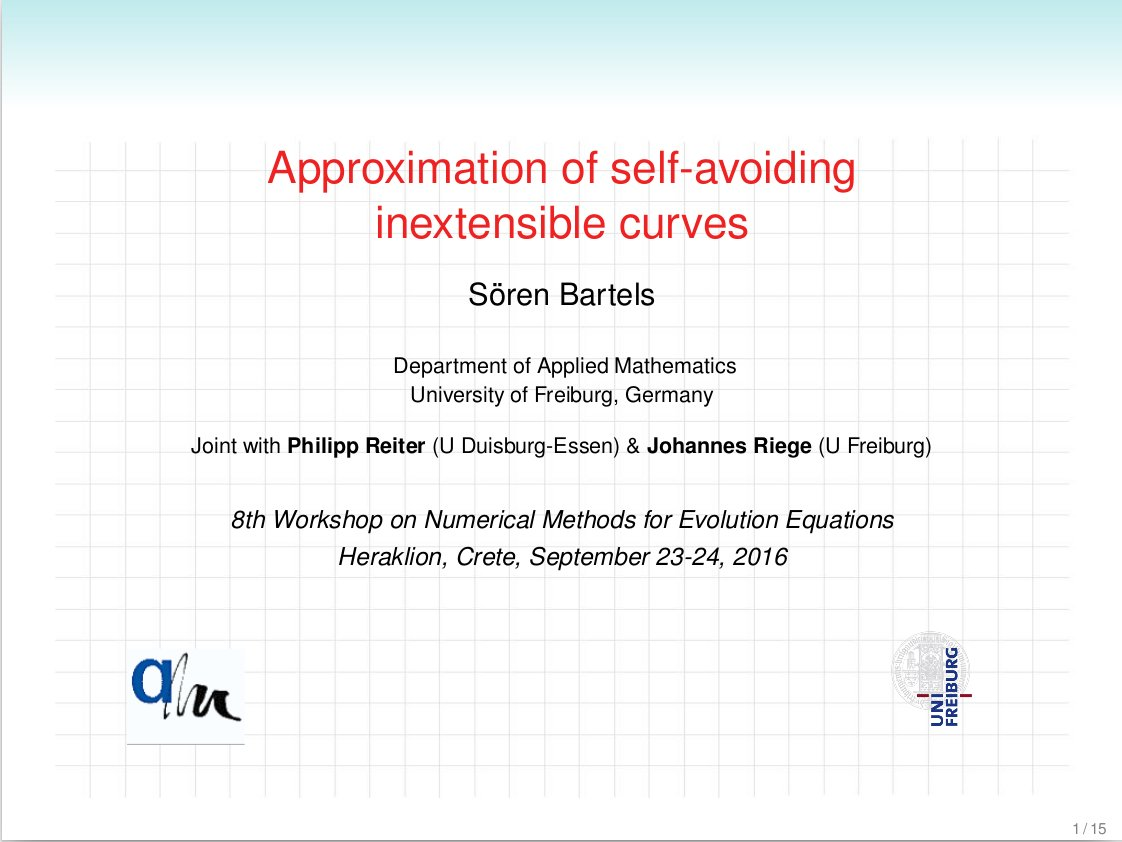# Ausgewählte VorträgeS. Bartels Paper Folding: Modeling and Simulation SFB 1481 Sparsity and Singular Structures Kickoff Workshop, Aachen September 22, 2022

Abstract. The folding of a thin elastic sheet along a curved arc has various applications including the construction of bistable devices. We discuss the derivation of a plate model from three-dimensional hyperelasticity and rigidity properties of admissible deformations and minimizers. The numerical solution is based on an isoparametric discontinuous Galerkin finite element method that provides a suitable geometric approximation of the folding arc. Error estimates are presented for a linearized version of the model problem. The talk is based on joint work with Andrea Bonito (Texas A&M), Peter Hornung (U Dresden), and Philipp Tscherner (U Freiburg).S. Bartels Simulation of Free Boundary Problems in the Nonlinear Bending of Elastic Rods and Plates 15th International Conference on Free Boundary Problems Online Conference, Berlin September 15, 2021

Abstract. The mathematical description of large bending deformations of thin elastic rods and plates leads to fourth order problems with nonlinear pointwise constraints that give rise to various free boundary phenomena. The free boundary may separate regions of trivial and large deformations, describe the contact zone in the presence of an obstacle, or be related to the occurrence of self-contact. We devise and analyse numerical methods that are capable of reliably capturing these effects under minimal regularity assumptions and which allow us to experimentally study topological transitions of free boundaries.S. Bartels et al. Error estimates for nonconforming and discontinuous discretizations of nonsmooth problems via convex duality Sayas Numerics Seminar Joint online seminar by UMD College Park & Baltimore, George Mason U, U Delaware September 7, 2021

Abstract. Nonconforming and discontinuous finite elements are attractive for discretising variational problems with limited regularity properties, in particular, when discontinuitiesmay occur. The possible lack of differentiability of related functionals prohibits the use of classical arguments to derive error estimates. As an alternative we make use of discrete andcontinuous convex duality relations and of quasi-interpolation operators with suitable projection properties. For total variation regularised minimisation problems a quasi-optimal error estimate is derived which is not available for standard finite element methods. Our results use and extend recent ideas by Chambolle and Pock.S. Bartels and P. Reiter Approximating gradient flow evolutions of self-avoiding inextensible curves and elastic knots Thematic Programme on Numerical analysis of complex PDE models in the sciences Erwin-Schrödinger-Institut, Vienna, Austria, July 18, 2018

Abstract. We discuss a semi-implict scheme that allows for minimizing the bending energy of curves within certain isotopy classes. To this end we consider a weighted sum of a bending energy and a tangent-point functional. The latter is defined via the iterated integral of a negative power of the tangent-point radius which is for a given pair of points the radius of the circle that is tangent to the curve in one point and intersects the curve in the other point. Evolutions are defined via gradient flows for the total energy within classes of arclength-parametrized curves. The numerical scheme is obtained by a semi-implicit discretization which treats the nonlinear and nonlocal tangent-point functional explicitly. This avoids the occurrence of fully populated matrices and allows for a straightforward parallelization of its computation. Based on estimates for the second derivative of the tangent-point functional and a uniform bi-Lipschitz radius, we prove a stability result implying energy decay during the evolution as well as maintenance of arclength parametrization. We present some numerical experiments exploring the energy landscape, targeted to the question how to obtain global minimizers of the bending energy in knot classes, so-called elastic knots.S. Bartels et al. Numerical solution of nonsmooth problems and application to damage evolution and optimal insulation Foundations of Computational Mathematics Barcelona, Spain, July 17, 2017

Abstract. Nonsmooth minimization problems and singular partial differential equations arise in the description of inelastic material behavior, image processing, and modeling of non-Newtonian fluids. The numerical discretization and iterative solution is often based on regularizing or stabilizing terms. In this talk we address the influence of such modifications on error estimates and the robustness of iterative solution methods. In particular, we present an unconditional stability result for semi-implicit discretizations of a class of singular flows and devise a variant of the alternating direction of multipliers method with variable step sizes. The results and methods are illustrated by numerical experiments for a damage evolution model and a problem of optimal insulation leading to a break of symmetry.S. Bartels, P. Reiter, J. Riege Approximation of self-avoiding inextensible curves 8th Workshop on Numerical Methods for Evolution Equations Heraklion, Crete, September 23, 2016

Abstract. The prediction of the behavior of elastic curves arises in knot theory and the modeling of filament networks in biological cells. When large deformations are considered or when one is interested in determining homotopy classes, injectivity of deformations has to be imposed. A practical realization consists in including an appropriate potential in the energy functional. Its numerical treatment is difficult due to the singular and nonlocal nature. We present a discretization of a tangent-point potential that leads to a simple and stable scheme and which allows for the use of large step sizes.S. Bartels, P. Schön Adaptive approximation of the Monge-Kantorovich problem Mafelap 2016 Brunel, UK, June 15, 2016

Abstract. Optimal transportation problems define high-dimensional linear programs. An efficient approach to their numerical solution is based on reformulations as nonlinear partial differential equations. If transportation cost is proportional to distance this leads to the Monge--Kantorovich problem which is a constrained minimization problem on Lipschitz continuous functions. We discuss the iterative solution via splitting methods and devise an adaptive mesh refinement strategy based on an a~posteriori error estimate for the primal-dual gap.